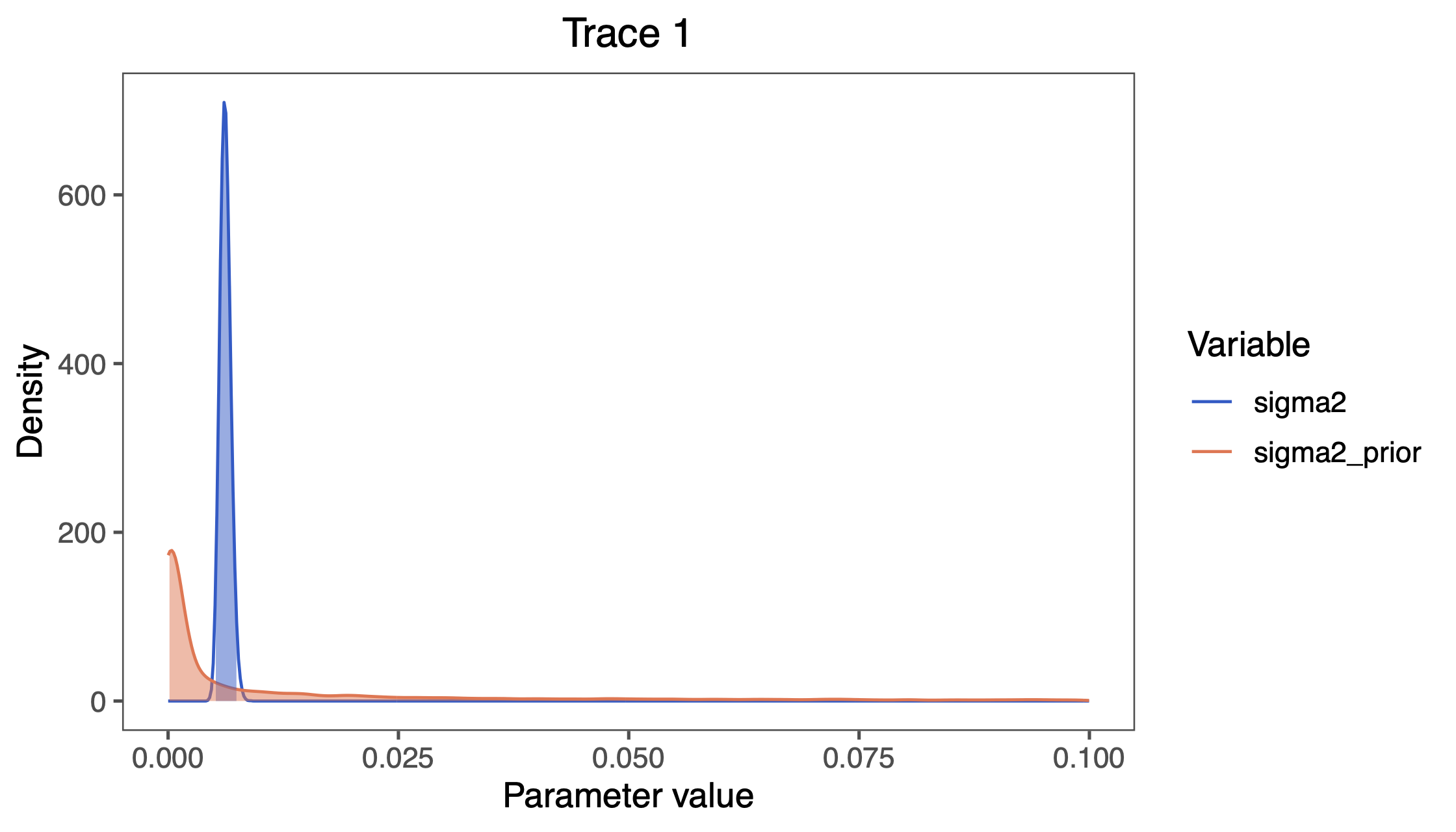# Simple Brownian Rate Estimation

## Estimating Constant Rates of Evolution

This tutorial demonstrates how to specify a Brownian-motion model where the rate of evolution is assumed to be constant among branches of a time-calibrated phylogeny (Felsenstein 1985) using the datasets of (log) body-size across vertebrate clades from (missing reference). We provide the probabilistic graphical model representation of each component for this tutorial. After specifying the model, you will estimate the rate of Brownian-motion evolution using Markov chain Monte Carlo (MCMC).

## Brownian-motion Model

Under the simple Brownian-motion (BM) model, the evolution of a continuous character is entirely determined by a single rate parameter, $\sigma^2$. The expected amount of change over a single branch of length $t$ is zero, and the variance in changes is $t \times \sigma^2$. We can estimate the posterior distribution of the rate parameter under this model by placing a prior on $\sigma^2$. The resulting graphical model is quite simple, as the probability of the continuous characters depends only on the phylogeny (which we assume to be known in this tutorial) and the rate parameter ().

For this exercise, we will specify a BM model, such that the rate parameter is a stochastic node, drawn from a Loguniform prior distribution, as depicted in . As we are specifying a Bayesian model, we focus on estimating the posterior distribution of the rate parameter given the continuous characters, $X$:

In this tutorial, we use the 66 vertebrate phylogenies and (log) body-size datasets from (Landis and Schraiber 2017).

⇨ The full BM-model specification is in the file called mcmc_BM.Rev.

We begin by deciding which of the 66 vertebrate datasets to use. Here, we assume we are analyzing the first dataset (Acanthuridae), but you should feel free to choose any of the datasets.

dataset <- 1


Now, we read in the (time-calibrated) tree corresponding to our chosen dataset.

T <- readTrees("data/trees.nex")[dataset]


Next, we read in the character data for the same dataset.

data <- readContinuousCharacterData("data/traits.nex")[dataset]


Additionally, we initialize a variable for our vector of moves and monitors:

moves    = VectorMoves()
monitors = VectorMonitors()


### Specifying the model

#### Tree model

In this tutorial, we assume the tree is known without area. We create a constant node for the tree that corresponds to the observed phylogeny.

tree <- T


#### Rate parameter

The constant-rate BM model has just one parameter, $\sigma^2$. We draw the rate parameter from a loguniform prior. This prior is uniform on the log scale, which means that it is represents ignorance about the order of magnitude of the rate.

sigma2 ~ dnLoguniform(1e-3, 1)


In order to estimate the posterior distribution of $\sigma^2$, we must provide an MCMC proposal mechanism that operates on this node. Because $\sigma^2$ is a rate parameter, and must therefore be positive, we use a scaling move called mvScale.

moves.append( mvScale(sigma2, weight=1.0) )


#### Brownian-motion model

Now that we have specified the parameters of the model, we can draw the character data from the corresponding phylogenetic Brownian-motion model. In this example, we use the REML algorithm to efficiently compute the likelihood (Felsenstein 1985). We provide the square root of the variance parameter, $\sigma$, to dnPhyloBrownianREML:

X ~ dnPhyloBrownianREML(tree, branchRates=sigma2^0.5)


Noting that $X$ is the observed data (), we clamp the data to this stochastic node.

X.clamp(data)


Finally, we create a workspace object for the entire model with model(). Remeber that workspace objects are initialized with the = operator, and are not themselves part of the Bayesian graphical model. The model() function traverses the entire model graph and finds all the nodes in the model that we specified. This object provides a convenient way to refer to the whole model object, rather than just a single DAG node.

mymodel = model(sigma2)


### Running an MCMC analysis

#### Specifying Monitors

For our MCMC analysis, we need to set up a vector of monitors to record the states of our Markov chain. The monitor functions are all called mn*, where * is the wildcard representing the monitor type. First, we will initialize the model monitor using the mnModel function. This creates a new monitor variable that will output the states for all model parameters when passed into a MCMC function.

monitors.append( mnModel(filename="output/simple_BM.log", printgen=10) )


Additionally, create a screen monitor that will report the states of specified variables to the screen with mnScreen:

monitors.append( mnScreen(printgen=1000, sigma2) )


#### Initializing and Running the MCMC Simulation

With a fully specified model, a set of monitors, and a set of moves, we can now set up the MCMC algorithm that will sample parameter values in proportion to their posterior probability. The mcmc() function will create our MCMC object:

mymcmc = mcmc(mymodel, monitors, moves, nruns=2, combine="mixed")


Now, run the MCMC:

mymcmc.run(generations=50000)


When the analysis is complete, you will have the monitored files in your output directory.

⇨ The Rev file for performing this analysis: mcmc_BM.Rev

### Exercise 1

• Run an MCMC simulation to estimate the posterior distribution of the BM rate (sigma).
• Load the generated output file into Tracer: What is the mean posterior estimate of sigma and what is the estimated HPD?
• Compare the prior mean with the posterior mean. (Hint: Use the optional argument underPrior=TRUE in the function mymcmc.run()) Are they different (e.g., )? Is the posterior mean outside the prior 95% probability interval?
• Repeat the analysis with a loguniform prior ranging from $1e-10$ to $1e10$.Estimates of the posterior and prior distribution of the sigma visualized in Tracer. The prior (green curve) shows the loguniform distribution that we chose as the prior distribution.
1. Felsenstein J. 1985. Phylogenies and the comparative method. The American Naturalist.:1–15. 10.1086/284325
2. Höhna S., Heath T.A., Boussau B., Landis M.J., Ronquist F., Huelsenbeck J.P. 2014. Probabilistic Graphical Model Representation in Phylogenetics. Systematic Biology. 63:753–771. 10.1093/sysbio/syu039
3. Landis M.J., Schraiber J.G. 2017. Pulsed evolution shaped modern vertebrate body sizes. Proceedings of the National Academy of Sciences. 114:13224–13229. 10.1073/pnas.1710920114